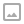## Send emails from Google sheet with app script

·   ·  ☕ 3 min read

### Section 1: Basic Setup

1. Create a new empty Spreadsheet.

2. Add a few rows of data. Every row should contain an email address in column A and the email message to be sent to that person in column B. For testing purposes, you may want to use your own email address in column A. Here’s an example:

3. Open the Script Editor by clicking on the Tools menu, then select Script editor.

4. Copy and paste the following script:

/**
* Sends emails with data from the current spreadsheet.
*/
function sendEmails() {
var startRow = 2; // First row of data to process
var numRows = 2; // Number of rows to process
// Fetch the range of cells A2:B3
var dataRange = sheet.getRange(startRow, 1, numRows, 2);
// Fetch values for each row in the Range.
var data = dataRange.getValues();
for (var i in data) {
var row = data[i];
var emailAddress = row; // First column
var message = row; // Second column
var subject = 'Sending emails from a Spreadsheet';
}
}

1. Save the Script.

2. Select the function sendEmails in the function dropdown list and click Run.

3. Check out your email inbox. Messages are usually immediately delivered, but sometimes it takes a few seconds.

4. You might want to have a look at the documentation for the following methods used in the script above:

Sheet.getRange() (note that there are four versions of this method)
Range.getValues()
MailApp.sendEmail() (note that there are four versions of this method)

### Section 2: Improvements

You might want to mark a cell in each row every time an email is sent. This way, if your script stops running (for instance, if there is a bug in your code or you reach the maximum number of emails you can send every minute or day) you will be able to re-run the script later on and avoid sending email duplicates.

Here’s a simple extension of the code that sets the cells in column C to ‘EMAIL_SENT’ for each row after sendEmail is called.

// This constant is written in column C for rows for which an email
// has been sent successfully.
var EMAIL_SENT = 'EMAIL_SENT';

/**
* Sends non-duplicate emails with data from the current spreadsheet.
*/
function sendEmails2() {
var startRow = 2; // First row of data to process
var numRows = 2; // Number of rows to process
// Fetch the range of cells A2:B3
var dataRange = sheet.getRange(startRow, 1, numRows, 3);
// Fetch values for each row in the Range.
var data = dataRange.getValues();
for (var i = 0; i < data.length; ++i) {
var row = data[i];
var emailAddress = row; // First column
var message = row; // Second column
var emailSent = row; // Third column
if (emailSent !== EMAIL_SENT) { // Prevents sending duplicates
var subject = 'Sending emails from a Spreadsheet';